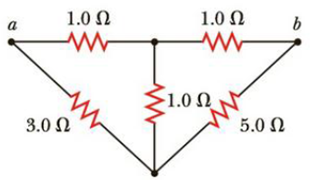Chapter 18, Problem 60AP

Chapter
Section
Textbook Problem

For the network in Figure P18.60, show that the resistance between points a and b is R a b = 27 17 Ω . (Hint: Connect a battery with emf ε across points a and b and determine ε/I, where I is the current in the battery.)Figure P18.60

(a)

To determine
The maximum electric power that the electric eel can generate.

Explanation

Given Info:

The total number of cells is 4.0×103 .

The potential difference of the each electrocyte is 150.0mV .

Explanation:

Formula to calculate the terminal potential difference is,

ΔV=N(ΔVe)

• ΔVe is the potential difference of each electrocyte
• N is the total number of cells

Substitute 4.0×103 for N and 150.0mV for ΔVe to find the total terminal potential difference,

ΔV=(4.0×103)(150.0×103V)=6.0×102V

Thus, the total terminal potential difference of cells is 6.0×102V

(b)

To determine
The energy released in one shock.

(c)

To determine
The height of a 1.0kg mass to be lifted so that its gravitational potential energy equals the energy released in 3.0×102 such shocks

Still sussing out bartleby?

Check out a sample textbook solution.

See a sample solution

The Solution to Your Study Problems

Bartleby provides explanations to thousands of textbook problems written by our experts, many with advanced degrees!

Get Started